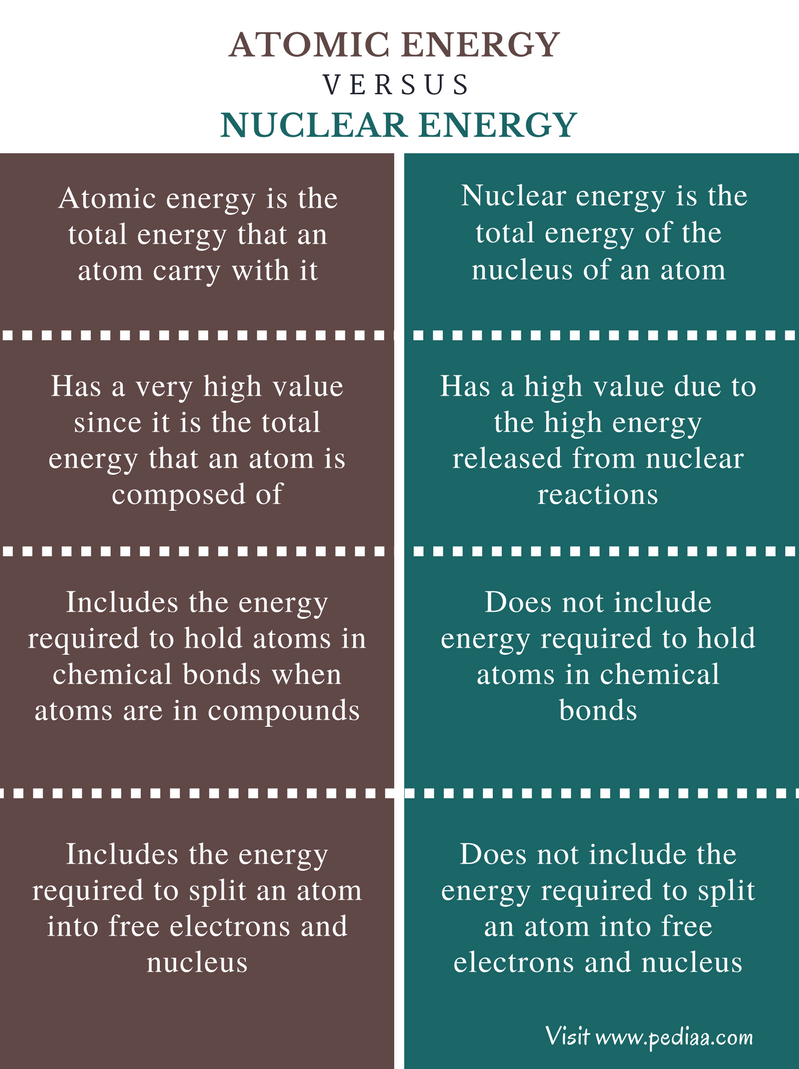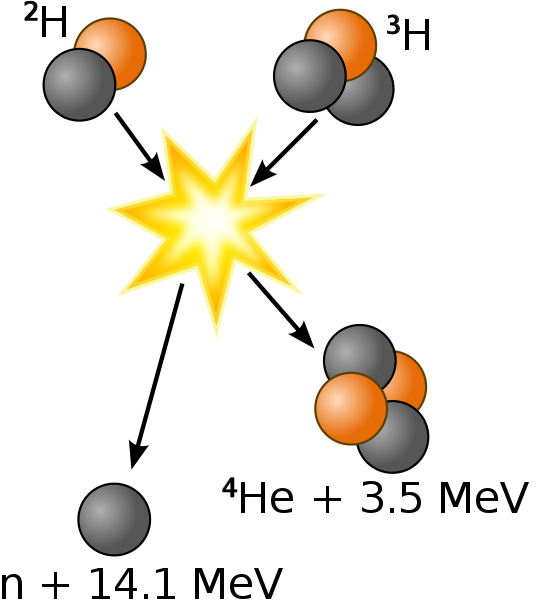# Difference Between Atomic Energy and Nuclear Energy

## Main Difference – Atomic Energy vs Nuclear Energy

All atoms are composed of a nucleus and an electron cloud around the nucleus. The nucleus is composed of protons and neutrons, which are subatomic particles. Each and every atom carries a certain amount of energy. This is called atomic energy. This atomic energy includes the potential energies of subatomic particles and the energy required to hold the electrons in orbitals around the nucleus. Nuclear energy refers to the energy released through fission and fusion of nucleus. The main difference between atomic energy and nuclear energy is that atomic energy includes energy required to hold electrons in an atom whereas nuclear energy does not include energy required to hold electrons

### Key Areas Covered

1. What is Atomic Energy
– Definition, Types, Examples
2. What is Nuclear Energy
– Definition, Types, Examples
3. What is the Difference Between Atomic Energy and Nuclear Energy
– Comparison of Key Differences

Key Terms: Atomic Energy, Atomic Binding Energy, Einstein Equation, Ionization Energy, Nuclear Binding Energy, Nuclear Fission, Nuclear Fusion, Neutrons, Nuclear Energy, Potential Energy, Radioactive Decay## What is Atomic Energy

Atomic energy is the total energy that an atom carries with it. The term atomic energy was first introduced before the discovery of nucleus. The atomic energy is the sum of different types of energies.

### Types of Energies

#### Atomic Binding Energy

The atomic binding energy of an atom is the energy required to disassemble an atom into free electrons and nucleus. It measures the energy required to remove electrons from the orbitals of an atom. This is also called the ionization energy when considering different elements.

#### Nuclear Binding Energy

This is the energy required to split the nucleus into neutrons and protons. In other words, nuclear binding energy is the energy that has been used to hold neutrons and protons together to form the nucleus. The binding energy is always a positive value since energy should be used in order to keep the forces between protons and neutrons.Figure 1: Nuclear binding energy of some elements

#### Potential Energy of the Nucleus

The potential energy is the sum of the potential energies of all the sub-atomic particle in a nucleus. Since the sub-atomic particles are not destroyed when a nuclear splitting is done, these particles will always have a potential energy. The potential energy can be converted into different energy forms.

#### Energy Released through Nuclear Fission and Fusion

Nuclear fission and nuclear fusion together can be called nuclear reactions. Nuclear fission is the process where a nucleus is split into smaller parts. Nuclear fusion is the process where two atomic nuclei combine to form a large single nucleus.

#### Energy Released in Radioactive Decay

Unstable nuclei undergo a special process called radioactive decay in order to obtain a stable state. There, neutrons or proton can be converted into different types of particles which are then emitted from the nucleus.

#### Energy of Atoms that are in Chemical Bonds

Compounds are composed of two or more atoms. These atoms are attached to each other through chemical bonds. In order to hold the atoms in these chemical bonds, a certain energy is required. This is called inter-atomic energy.

## What is Nuclear Energy

Nuclear energy is the total energy of the nucleus of an atom. Nuclear energy is released when nuclear reactions occur. Nuclear reactions are reactions that can change the nucleus of an atom. There are two major types of nuclear reactions as nuclear fission reactions and nuclear fusion reactions.

### Nuclear Fission

A nuclear fission is the splitting of nucleus into smaller particles. These particles are called fission products. When a nuclear fission occurs, the final total mass of fission products is not equal to the total initial mass of the nucleus. The final value is also less than the initial value. The missing mass is converted into energy. The energy released can be found using the Einstein equation.

#### E     =     mc2

Where E is the energy released, m is the missing mass and c is the speed of the light.

A nuclear fission can occur in three ways:

Radioactive decay occurs in unstable nuclei. Here, some sub-atomic particles are converted into different forms of particles and are emitted spontaneously. This occurs in order to obtain a stable state.

### Neutron Bombardment

Nuclear fission can occur through neutron bombardment. When a nucleus is hit with a neutron from the outside, the nucleus may split into fragments. These fragments are called fission products. This releases a high amount of energy along with more neutrons of the nucleus.

### Nuclear Fusion

Nuclear fission takes place when two or more nuclei combine with each other forming a new single nucleus. A large amount of energy is released here. The missing mass during the process of fusion is converted into energy.Figure 2: Nuclear Fusion Reaction

The above examples shows the fusion of Deuterium (2H) and Tritium (3H). The reaction gives Helium (4He) as the end product along with a neutron. The reaction yields a total of 17.6 MeV.

Nuclear energy is a good source of energy for electricity production. Nuclear power reactors are capable of utilizing nuclear energy in order to produce electricity. The energy density of elements that can be used in nuclear reactors is very high compared to other energy sources such as fossil fuels. However, a major drawback of nuclear energy usage is the formation of nuclear waste and dramatic accidents that can occur in power plants.

## Difference Between Atomic Energy and Nuclear Energy

### Definition

Atomic Energy: Atomic energy is the total energy that an atom carries with it.

Nuclear Energy: Nuclear energy is the total energy of the nucleus of an atom.

### Value

Atomic Energy: The atomic energy has a very high value since it is the total energy that an atom is composed of.

Nuclear Energy: Nuclear energy is a high value due to the high energy released from nuclear reactions.

### Chemical Bonding

Atomic Energy: Atomic energy includes the energy required to hold atoms in chemical bonds when atoms are in compounds.

Nuclear Energy: Nuclear energy does not include energy required to hold atoms in chemical bonds

### Electrons

Atomic Energy: Atomic energy includes the energy required to split an atom into free electrons and nucleus.

Nuclear Energy: Nuclear energy does not include the energy required to split an atom into free electrons and nucleus.

### Conclusion

Both atomic energy and nuclear energy are defined regarding atoms. Atomic energy includes the sum of the energy included in an atom. Nuclear energy includes the energy released when changes are done to the nucleus of an atom. This the main difference between atomic energy and nuclear energy.

##### Reference:

1.”Nuclear Fusion.” Atomci Archive.National Science Digital Library, n.d. Web. Available here. 28 July 2017.
2.”Nuclear Fusion.” Nuclear Fusion. N.p., n.d. Web. Available here. 28 July 2017.

##### Image Courtesy:

“Binding energy curve – common isotopes” (Public Domain) via Commons Wikimedia
“Deuterium-tritium fusion” By Wykis – Own work, based on w:File:D-t-fusion.png (Public Domain) via Commons Wikimedia